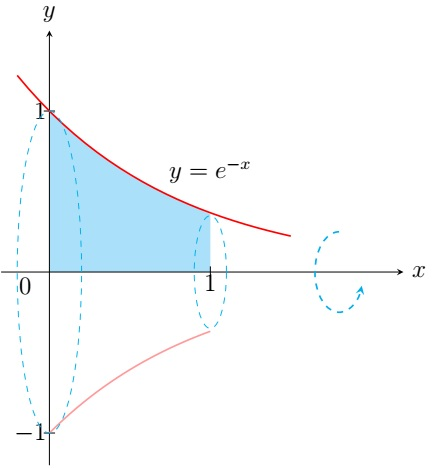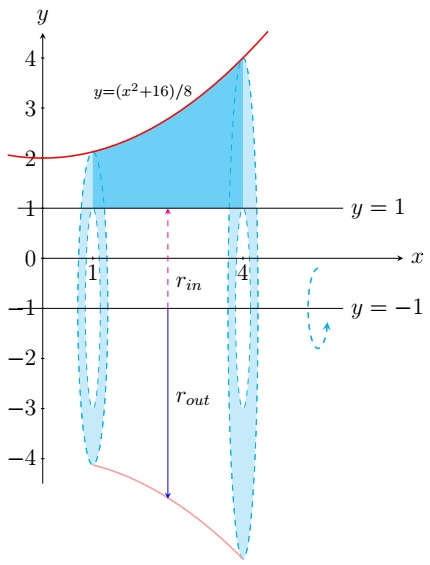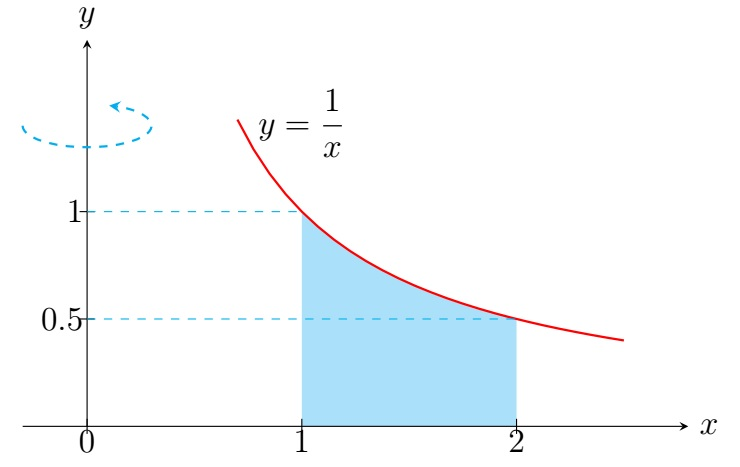Calculus II Home

## Volume of a Solid of Revolution

A solid $$S$$ of revolution is obtained by rotating a region about a line. First suppose $$S$$ is obtained by rotating a region about the $$x$$-axis or a line on the $$xy$$-plane parallel to the $$x$$-axis. If $$S$$ is between $$x=a$$ and $$x=b$$ such that $$A(x)$$ is the area of its vertical cross-section at $$x$$ and $$A$$ is a continuous function on $$[a,b]$$, then the volume of $$S$$ is $V(S)= \int_a^b A(x)\;dx.$ Disk Method: If the vertical cross-section is a disk with radius $$r(x)$$, then $$A(x)=\pi r^2$$ and $V(S)= \int_a^b \pi r^2 \;dx.$ Washer Method: If the vertical cross-section is a washer (annular ring) with the inner radius $$r_{in}(x)$$ and the outer radius $$r_{out}(x)$$, then $$A(x)=\pi \left( r_{out}^2-r_{in}^2 \right)$$ and $V(S)= \int_a^b \pi \left( r_{out}^2-r_{in}^2 \right) \;dx.$

Example. Find the volume of the solid obtained by rotating the region bounded by $$y=e^{-x}$$, $$x=0$$, $$x=1$$, and $$y=0$$ about the $$x$$-axis.

Solution. The cross-section of the solid at $$x$$ perpendicular to the $$x$$-axis is a disk with the radius $$e^{-x}$$ and the area $$\pi \left( e^{-x}\right)^2=\pi e^{-2x}$$. Since the solid is in between $$x=0$$ and $$x=1$$, its volume is $\int_0^1 \pi e^{-2x} \;dx= \left.\pi \frac{e^{-2x}}{-2}\right\vert_0^1=-\frac{\pi}{2} \left( e^{-2}-e^0 \right)=\frac{\pi\left(e^2-1 \right)}{2e^2}.$Example. Find the volume of the solid obtained by rotating the region bounded by $$y=\frac{x^2+16}{8}$$, $$y=1$$, $$x=1$$, and $$x=4$$ about the line $$y=-1$$.

Solution. The cross-section of the solid at $$x$$ perpendicular to the $$x$$-axis is a washer with the inner radius $$r_{in}=1-(-1)=2$$ and the outer radius $$r_{out}=\frac{x^2+16}{8}-(-1)=\frac{x^2}{8}+3$$. Since the solid is in between $$x=1$$ and $$x=4$$, its volume is \begin{align*} \int_1^4 \pi \left( r_{out}^2-r_{in}^2 \right) \;dx &= \int_1^4 \pi \left( \left(\frac{x^2}{8}+3\right)^2-2^2 \right) \;dx\\ &= \int_1^4 \pi \left( \frac{x^4}{64}+\frac{3}{4}x^2+5 \right) \;dx\\ &= \int_1^4 \frac{\pi}{64} \left( x^4+48x^2+320 \right) \;dx\\ &=\left.\frac{\pi}{64} \left( \frac{x^5}{5}+16x^3+320x \right) \right\vert_1^4\\ &=\frac{\pi}{64} \left[\left( \frac{4^5}{5}+16\cdot 4^3+320\cdot 4 \right)- \left( \frac{1^5}{5}+16\cdot 1^3+320\cdot 1 \right)\right]\\ &=\frac{10863 \pi}{320}. \end{align*}The above techniques will be similarly applied to a solid $$S$$ obtained by rotating a region about the $$y$$-axis or a line on the $$xy$$-plane parallel to the $$y$$-axis:
If $$S$$ is between $$y=c$$ and $$y=d$$ such that $$A(y)$$ is the area of its vertical cross-section at $$y$$ and $$A$$ is a continuous function on $$[c,d]$$, then the volume of $$S$$ is $V(S)= \int_c^d A(y)\;dy.$ We apply the disk method and the washer method similarly to find $$A(y)$$.

Example. Find the volume of the solid obtained by rotating the region bounded by $$xy=1$$, $$x=1$$, $$x=2$$, and $$y=0$$ about the $$y$$-axis.

Solution. The cross-section of the solid at $$y$$ perpendicular to the $$y$$-axis is a washer with the inner radius $$r_{in}=1$$ and the outer radius $$r_{out}=2$$ when $$0\leq y\leq 0.5$$ and $$r_{out}=\frac{1}{y}$$ when $$0.5\leq y\leq 1$$. Since the solid is in between $$y=0$$ and $$y=1$$, its volume is \begin{align*} \int_0^1 \pi \left( r_{out}^2-r_{in}^2 \right) \;dy &= \int_0^{0.5} \pi \left( 2^2-1^2 \right) \;dy +\int_{0.5}^1 \pi \left( \left( \frac{1}{y}\right)^2-1^2 \right) \;dy\\ &= \int_0^{0.5} 3\pi \;dy +\int_{0.5}^1 \pi \left( y^{-2}-1 \right) \;dy\\ &=\left.3\pi y \right\vert_0^{0.5} +\left. \pi\left( -\frac{1}{y}-y \right) \right\vert_{0.5}^1\\ &=3\pi \cdot 0.5+ \pi\left[\left( -1-1 \right)- \left( -\frac{1}{0.5}-0.5\right)\right]\\ &=1.5\pi +0.5\pi\\ &=2\pi. \end{align*}Last edited